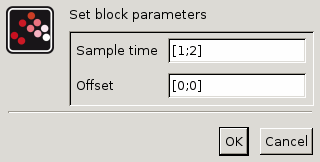Scilab Home page | Wiki | Bug tracker | Forge | Mailing list archives | ATOMS | File exchange
Change language to: Français - Português - 日本語 - Русский

See the recommended documentation of this function

Scilab Help >> Xcos > palettes > Event handling palette > M_freq

# M_freq

Multiple Frequencies

### Block Screenshot### Description

This blocks generates events at specific sample time of the simulation time. The sample time is given in the "Sample Time" field and the offset is given in the "Offset" field. This block has one event input, the number of event outputs depends on the number of different sample time. For example if the vector of sample time is [1 1 2] and the vector of offset is [0 .5 0] then the block has 7 outputs.

- The first output is activated when the simulation time is equal to a multiple of the first sample time plus the first offset

- The second output is activated when the simulation time is equal to a multiple of the second sample time plus the second offset.

- The third output is activated when we have both cases, first case and second case.

- The fourth output is activated when the simulation time is equal to a multiple of the third sample time plus the third offset.

- The fifth output is activated when we have both cases, first case and forth case.

- The sixth output is activated when we have both cases, second case and fourth case.

- The seventh output is activated when we have both cases, third case and forth case.

etc...

So the number of outputs is equal to 2**number of different time values. Each of these time values is represented by a binary number associated to the output's number in decimal.

### Dialog box• Sample time

Vector of sample time values.

Properties : Type 'vec' of size -1.

• Offset

Vector of offset values. Must have the same size as the Sample time and each offset value must be less than its corresponding sample time.

Properties : Type 'vec' of size -1.

### Example

```Let us take the example where the sample time is equal to [1 1 2] and the offset is equal to [0 .5 0]. Consider t=simulation time.
When t=0, the fifth output is activated (001 + 100).
When t=0.5, the second output is activated (010).
When t=1, the first output is activated (001).
When t=1.5, the second output is activated (010).
When t=2 we loop back to 0.```

### Default properties

• always active: no

• direct-feedthrough: no

• zero-crossing: no

• mode: no

• number/sizes of activation inputs: 1

• number/sizes of activation outputs: 3

• continuous-time state: no

• discrete-time state: no

• object discrete-time state: no

• name of computational function: m_frequ

### Interfacing function

• SCI/modules/scicos_blocks/macros/Events/M_freq.sci

### Computational function

• SCI/modules/scicos_blocks/src/c/m_frequ.c (Type 4)

### Multiple frequency example